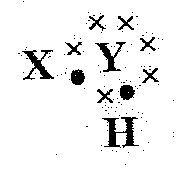# AP Tenth Class Physical Science Chemical Bonding(EM) Important Questions

1 MARKS QUESTIONS

8. CHEMICAL BONDING

1.     Why does it is difficult to remove an electron from Mg+ ion than neutral Mg atom?

2.     What is the valency of the element having atomic number 20?

3.     The electron configuration of an atom is 2, 8, 7. Whether it forms anion or cation? Why?

4.     Represent each of the following atoms using Lewis notation:

a. Beryllium        b. Calcium          c. Lithium

5.     Represent the molecule H2o using Lewis notation.

2 MARKS QUESTIONS

1.     Why does Sodium always form a cation where as Fluorine atom always forms an anion?

2.     Give the reason for low melting point for covalent compound when compared with ionic compounds?

3.     What is octet rule? How do you appreciate role of the octet rule' in explaining the chemical properties of elements?

4.     A chemical compound has the following Lewis notation:a. How many valence electrons does element Y have?

b. What is the valence of element Y?

c. What is the valence of element X?

d. How many covalent bonds are in the molecule

e. Suggest a name for the elements X and Y.

5.     What is hybridization? Explain the formation of the following molecules using hybridization.

a) Be Cl2            b) BF3 ​​​​​​​

4 MARKS QUESTIONS

1.     Collect the information about properties and uses of covalent compounds and prepare a report?

2.     Draw simple diagrams to show how electrons are arranged in the following covalent molecules:

a) Calcium oxide (Cao) (b) Water (H2o) (C) Chlorine (Cl2

3.     Explain the formation of the following molecules using valence bond theory.

a) Formation of N2 molecule.

b) Formation of O2 molecule.

4.     Explain the formation of sodium chloride and calcium oxide on the basis of the concept of electron transfer from one atom to another atom.

Published date : 29 Apr 2022 07:09PM